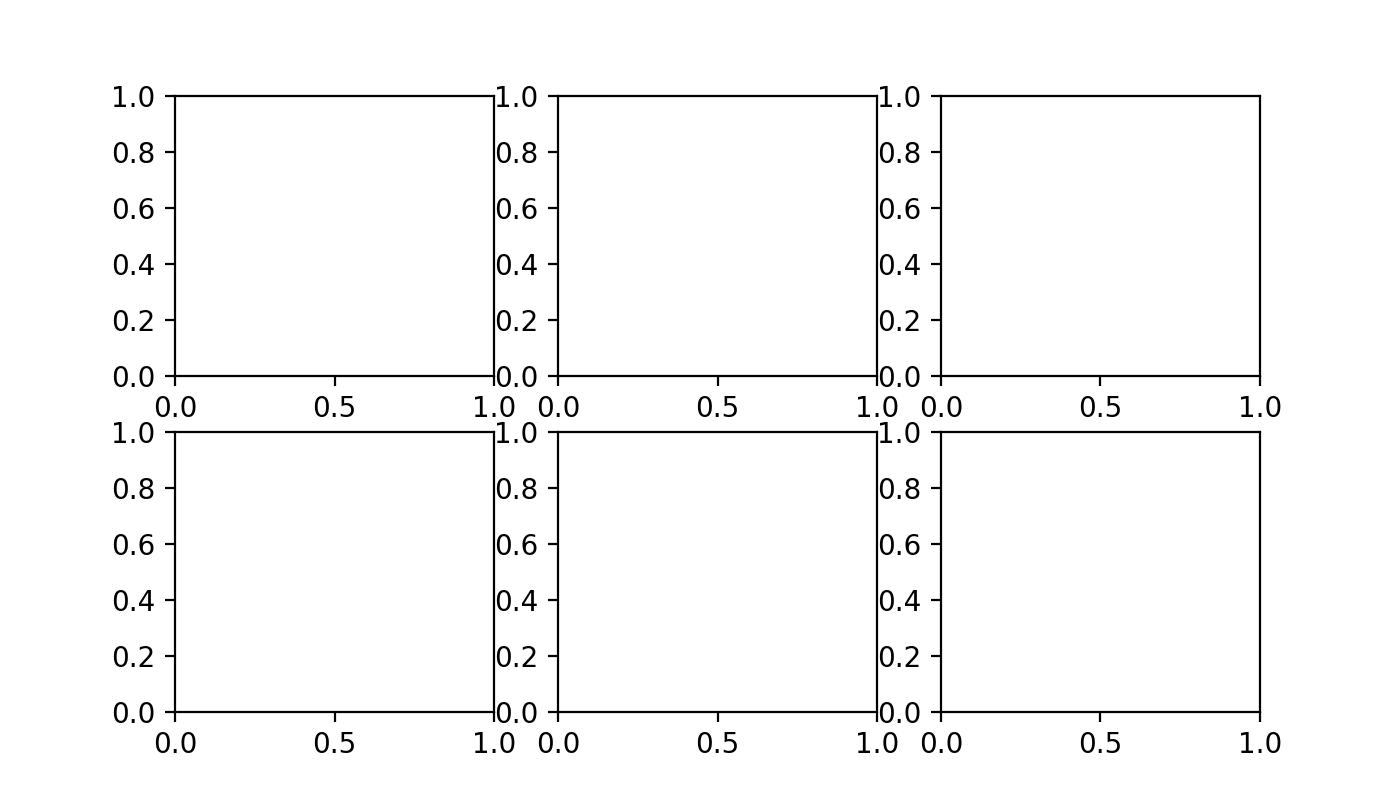# Dates vs Derivatives Solution¶

## Solution 1 (Object Oriented)¶

import matplotlib.pyplot as plt

# Instantiate a figure and an Axes
fig, ax = plt.subplots()

# Draw a scatter plot on the axes
ax.plot(derivatives, dates, c="tab:red", linestyle='None', marker='o')

# Draw the title and labels
ax.set_title("Dates vs Derivatives\n(for 30 college students surveyed about their recent year)")
ax.set_xlabel("derivates")
ax.set_ylabel("dates")


### Explanation¶

1. Make a figure with an Axes.

fig, ax = plt.subplots()


pyplot.subplots() is a handy function for creating a figure and a set of subplots in a single line. The default behavior creates a single figure with a single Axes, but if we wanted, we could make a grid of Axes with something like this.

fig, ax = plt.subplots(nrows=3, ncols=3)Alternatively, we could create the figure and axes in a less cryptic (but lengthier) way, like this.

fig = plt.figure()  # make the figure
ax = fig.axes    # get the Axes object (for downstream changes)


Note

Note that ax is technically an AxesSubplot.

type(ax)
# <class 'matplotlib.axes._subplots.AxesSubplot'>


AxesSubplot is a subclass of the more generic Axes class.

2. Draw a scatter plot on the Axes.

ax.plot(derivatives, dates, c="tab:red", linestyle='None', marker='o')


Here we use the Axes.plot() method, passing in derivatives, dates, c="tab:red" (color), linestyle='None', and marker='o'. By default, Axes.plot() connects points with lines (i.e. it draws a line plot). By setting linestyle='None' and marker='o', we get a scatter plot.

What about Axes.scatter()

It's true, ax.scatter(x=derivatives, y=dates, c="tab:red") works, but it's less performant than ax.plot(x=derivatives, y=dates, c="tab:red", linestyle='None').

Use Axes.scatter() when you want to customize the points (e.g. varying their sizes or colors by another variable).

Tip

"tab:red" stands for Tableau red. See the Tableau color palette here.

You can find a list of additional matplotlib color palettes here.

3. Draw the title and labels.

title = "Dates vs Derivatives\n(for 30 college students surveyed about their recent year)"
ax.set_title(title)        # (1)!
ax.set_xlabel("derivates") # (2)!
ax.set_ylabel("dates")     # (3)!


## Solution 2 (PyPlot)¶

# Make a scatter plot
plt.scatter(x=derivatives, y=dates, c="tab:red")

# Draw the title and labels
plt.title("Dates vs Derivatives\n(for 30 college students surveyed about their recent year)")
plt.xlabel("derivates")
plt.ylabel("dates")


### Explanation¶

This solution uses the matplotlib.pyplot state-based interface to matplotlib, with methods:

Is this better than Solution 1?

No.

Solution 1 is preferred because it provides more control over the figure and axes objects. ..but this solution is perfectly fine for ad hoc analyses.